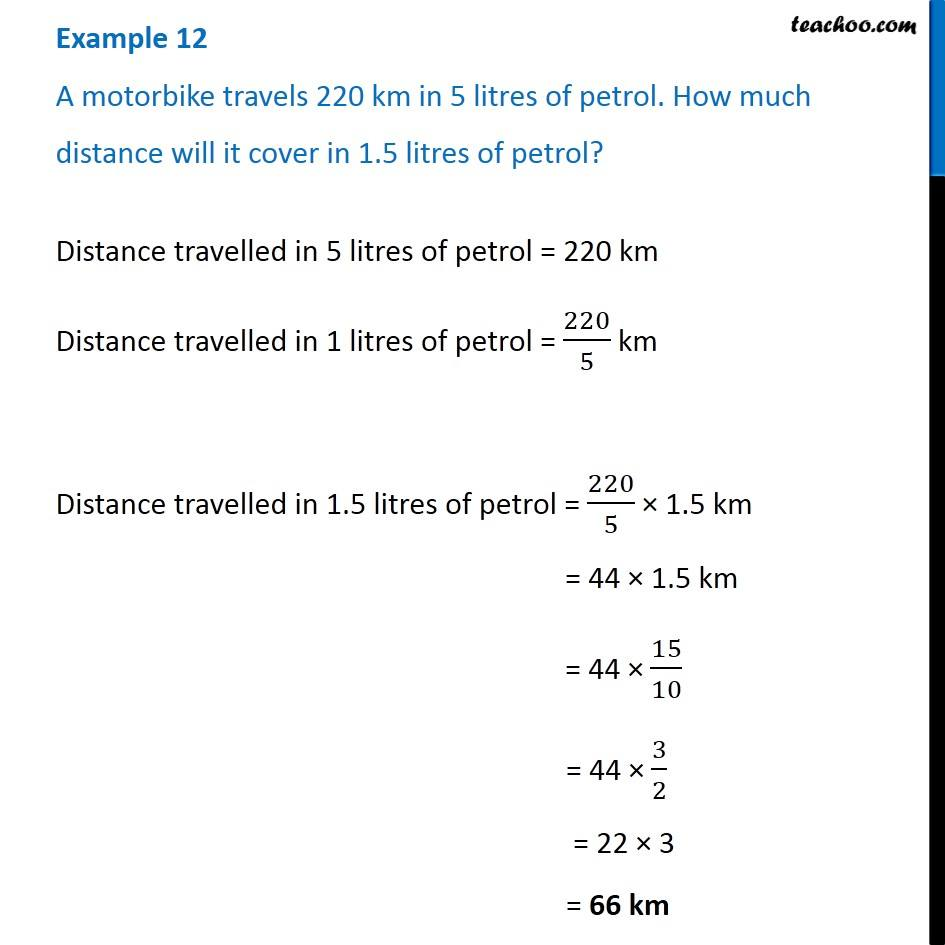Subscribe to our Youtube Channel - https://you.tube/teachoo

1. Chapter 12 Class 6 Ratio And Proportion
2. Concept wise
3. Unitary Method

Transcript

Example 12 A motorbike travels 220 km in 5 litres of petrol. How much distance will it cover in 1.5 litres of petrol?Distance travelled in 5 litres of petrol = 220 km Distance travelled in 1 litres of petrol = 220/5 km Distance travelled in 1.5 litres of petrol = 220/5 × 1.5 km = 44 × 1.5 km = 44 × 15/10 = 44 × 3/2 = 22 × 3 = 66 km

Unitary Method